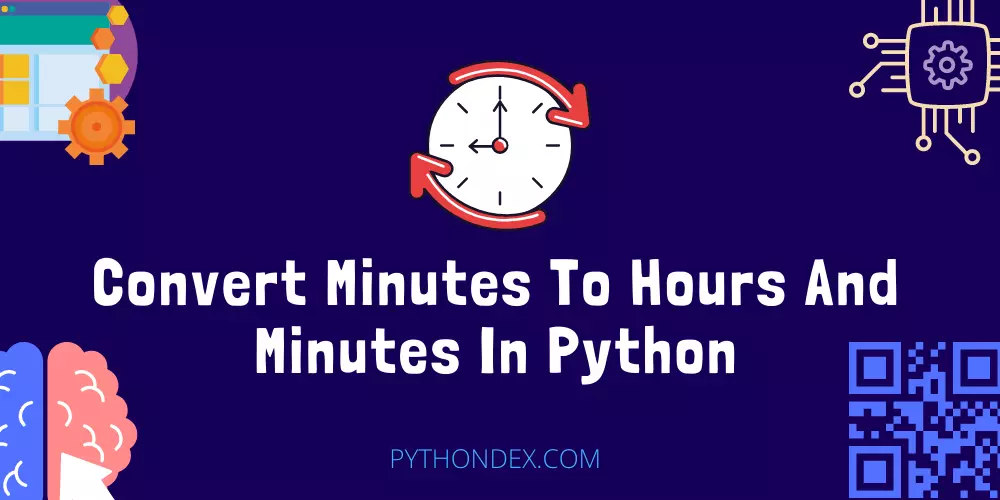ExplorePython Programs# Convert Minutes To Hours And Minutes In PythonLooking for a tutorial on python convert minutes to hours and minutes then you are at the right place, today in this python tutorial I will show you how to convert minutes to hours and minutes in python.

Before I show you how to convert minutes to hours in python, let’s see what do we mean by it and how this will work

``````
# Example 1
INPUT: 120 Minutes
OUTPUT: 2 hours

# Example 2
INPUT: 220 Minutes
OUTPUT: 3:40 hours
```
```

The program should convert the given minutes in hours and minutes format like 110 minutes will be 1:50 hours, so now let’s convert this in python code.

## Convert Minutes To Hours And Minutes In Python Code

``````
total_minutes = 130

# Get hours with floor division
hours = total_minutes // 60

# Get additional minutes with modulus
minutes = total_minutes % 60

# Create time as a string
time = "{}:{}".format(hours, minutes)

print(time)
```
```

Above is the python code to convert minutes to hours and minutes format copy and paste this code in a python file and run it in your python compiler or use an online compiler.

After you run the above code, below is the output you should get, you can add any minutes in the minutes variable

``````
2:10
```
```

If you run the program successfully which should, then you can see that it converts the minutes into hours and minutes format.

## Summary

So this was the tutorial to convert minutes to hours and minutes in python. I hope you successfully ran this program and found what you were looking for.

Here are more python guides you may find helpful: